Open in App
Not now

# Student record management system using linked list

• Difficulty Level : Easy
• Last Updated : 16 Dec, 2022

Problem: Create a student Record Management system that can perform the following operations:

• Insert Student record
• Delete student record
• Show student record
• Search student record

The student record should contain the following items

• Name of Student
• Roll Number of Student
• Course in which Student is Enrolled
• Total Marks of Student

Approach: With the basic knowledge of operations on Linked Lists like insertion, deletion of elements in the Linked list, the student record management system can be created. Below are the functionalities explained that are to be implemented:

Exception Handling

Although the implementation of exception handling is quite simple few things must be taken into consideration before designing such a system:

• Roll Number must be used as a key to distinguish between two different records so while inserting a record check whether this record already exists in our database or not if it already exists then immediately report to the user that the record already exists and insert that record in the database.
• The record should be inserted in sorted order for this to make the roll number a key and use the inserting node in the sorted linked list.

Below is the implementation of the above approach:

## C++

 `// C++ program for the above approach``#include ``using` `namespace` `std;` `// Node Class``class` `Node {``public``:``    ``int` `roll;``    ``string Name;``    ``string Dept;``    ``int` `Marks;``    ``Node* next;``};` `// Stores the head of the Linked List``Node* head = ``new` `Node();` `// Check Function to check that if``// Record Already Exist or Not``bool` `check(``int` `x)``{``    ``// Base Case``    ``if` `(head == NULL)``        ``return` `false``;` `    ``Node* t = ``new` `Node;``    ``t = head;` `    ``// Traverse the Linked List``    ``while` `(t != NULL) {``        ``if` `(t->roll == x)``            ``return` `true``;``        ``t = t->next;``    ``}` `    ``return` `false``;``}` `// Function to insert the record``void` `Insert_Record(``int` `roll, string Name,``                   ``string Dept, ``int` `Marks)``{``    ``// if Record Already Exist``    ``if` `(check(roll)) {``        ``cout << ``"Student with this "``             ``<< ``"record Already Exists\n"``;``        ``return``;``    ``}` `    ``// Create new Node to Insert Record``    ``Node* t = ``new` `Node();``    ``t->roll = roll;``    ``t->Name = Name;``    ``t->Dept = Dept;``    ``t->Marks = Marks;``    ``t->next = NULL;` `    ``// Insert at Begin``    ``if` `(head == NULL``        ``|| (head->roll >= t->roll)) {``        ``t->next = head;``        ``head = t;``    ``}` `    ``// Insert at middle or End``    ``else` `{``        ``Node* c = head;``        ``while` `(c->next != NULL``               ``&& c->next->roll < t->roll) {``            ``c = c->next;``        ``}``        ``t->next = c->next;``        ``c->next = t;``    ``}` `    ``cout << ``"Record Inserted "``         ``<< ``"Successfully\n"``;``}` `// Function to search record for any``// students Record with roll number``void` `Search_Record(``int` `roll)``{``    ``// if head is NULL``    ``if` `(!head) {``        ``cout << ``"No such Record "``             ``<< ``"Available\n"``;``        ``return``;``    ``}` `    ``// Otherwise``    ``else` `{``        ``Node* p = head;``        ``while` `(p) {``            ``if` `(p->roll == roll) {``                ``cout << ``"Roll Number\t"``                     ``<< p->roll << endl;``                ``cout << ``"Name\t\t"``                     ``<< p->Name << endl;``                ``cout << ``"Department\t"``                     ``<< p->Dept << endl;``                ``cout << ``"Marks\t\t"``                     ``<< p->Marks << endl;``                ``return``;``            ``}``            ``p = p->next;``        ``}` `        ``if` `(p == NULL)``            ``cout << ``"No such Record "``                 ``<< ``"Available\n"``;``    ``}``}` `// Function to delete record students``// record with given roll number``// if it exist``int` `Delete_Record(``int` `roll)``{``    ``Node* t = head;``    ``Node* p = NULL;` `    ``// Deletion at Begin``    ``if` `(t != NULL``        ``&& t->roll == roll) {``        ``head = t->next;``        ``delete` `t;` `        ``cout << ``"Record Deleted "``             ``<< ``"Successfully\n"``;``        ``return` `0;``    ``}` `    ``// Deletion Other than Begin``    ``while` `(t != NULL && t->roll != roll) {``        ``p = t;``        ``t = t->next;``    ``}``    ``if` `(t == NULL) {``        ``cout << ``"Record does not Exist\n"``;``        ``return` `-1;``        ``p->next = t->next;` `        ``delete` `t;``        ``cout << ``"Record Deleted "``             ``<< ``"Successfully\n"``;` `        ``return` `0;``    ``}``}` `// Function to display the Student's``// Record``void` `Show_Record()``{``    ``Node* p = head;``    ``if` `(p == NULL) {``        ``cout << ``"No Record "``             ``<< ``"Available\n"``;``    ``}``    ``else` `{``        ``cout << ``"Index\tName\tCourse"``             ``<< ``"\tMarks\n"``;` `        ``// Until p is not NULL``        ``while` `(p != NULL) {``            ``cout << p->roll << ``"    \t"``                 ``<< p->Name << ``"\t"``                 ``<< p->Dept << ``"\t"``                 ``<< p->Marks << endl;``            ``p = p->next;``        ``}``    ``}``}` `// Driver code``int` `main()``{``    ``head = NULL;``    ``string Name, Course;``    ``int` `Roll, Marks;` `    ``// Menu-driven program``    ``while` `(``true``) {``        ``cout << ``"\n\t\tWelcome to Student Record "``                ``"Management System\n\n\tPress\n\t1 to "``                ``"create a new Record\n\t2 to delete a "``                ``"student record\n\t3 to Search a Student "``                ``"Record\n\t4 to view all students "``                ``"record\n\t5 to Exit\n"``;``        ``cout << ``"\nEnter your Choice\n"``;``        ``int` `Choice;` `        ``// Enter Choice``        ``cin >> Choice;``        ``if` `(Choice == 1) {``            ``cout << ``"Enter Name of Student\n"``;``            ``cin >> Name;``            ``cout << ``"Enter Roll Number of Student\n"``;``            ``cin >> Roll;``            ``cout << ``"Enter Course of Student \n"``;``            ``cin >> Course;``            ``cout << ``"Enter Total Marks of Student\n"``;``            ``cin >> Marks;``            ``Insert_Record(Roll, Name, Course, Marks);``        ``}``        ``else` `if` `(Choice == 2) {``            ``cout << ``"Enter Roll Number of Student whose "``                    ``"record is to be deleted\n"``;``            ``cin >> Roll;``            ``Delete_Record(Roll);``        ``}``        ``else` `if` `(Choice == 3) {``            ``cout << ``"Enter Roll Number of Student whose "``                    ``"record you want to Search\n"``;``            ``cin >> Roll;``            ``Search_Record(Roll);``        ``}``        ``else` `if` `(Choice == 4) {``            ``Show_Record();``        ``}``        ``else` `if` `(Choice == 5) {``            ``exit``(0);``        ``}``        ``else` `{``            ``cout << ``"Invalid Choice "``                 ``<< ``"Try Again\n"``;``        ``}``    ``}``    ``return` `0;``}`

## C

 `/* P3.1 Program of single linked list of non integer type node*/` `#include``#include``#include``#include``typedef` `struct` `student``{``    ``int` `roll_no;``    ``char` `name;``    ``float` `marks;``    ``struct` `student *next;``}node;``node *create_list();``void` `display(node *);``void` `count(node *);``void` `search(node *,``int``);``node *addatbeg(node *);``node *addatend(node *);``node *addafter(node *,``int``);``node *addbefore(node *,``int``);``node *addatpos(node *,``int``);``node *del(node *,``int``);``node *reverse(node *);` `void` `main(){``    ``int` `choice,data,item,pos;``    ``node *start;``    ``start=NULL;``    ``do``    ``{``        ``printf``(``"ENTER YOUR CHOICE\n"``);``        ``printf``(``"1.Create List\n"``);``        ``printf``(``"2.Display\n"``);``        ``printf``(``"3.Count\n"``);``        ``printf``(``"4.Search\n"``);``        ``printf``(``"5.Add to empty list / Add at beginning\n"``);``        ``printf``(``"6.Add at end\n"``);``        ``printf``(``"7.Add after node\n"``);``        ``printf``(``"8.Add before node\n"``);``        ``printf``(``"9.Add at position\n"``);``        ``printf``(``"10.Delete\n"``);``        ``printf``(``"11.Reverse\n"``);``        ``printf``(``"12.Quit\n\n"``);``        ` `        ``printf``(``"Enter your choice : "``);``        ``scanf``(``"%d"``,&choice);``        ` `        ``switch``(choice)``        ``{``         ``case` `1:``            ``start=create_list();``            ``break``;``         ``case` `2:``            ``display(start);``            ``break``;``         ``case` `3:``            ``count(start);``            ``break``;``         ``case` `4:``            ``printf``(``"Enter the element to be searched : "``);``            ``scanf``(``"%d"``,&data);``            ``search(start,data);``            ``break``;``        ``case` `5:``            ``start=addatbeg(start);``            ``break``;``         ``case` `6:``            ``start=addatend(start);``            ``break``;``         ``case` `7:``            ``printf``(``"Enter the roll no after which to insert : "``);``            ``scanf``(``"%d"``,&item);``            ``start=addafter(start,item);``            ``break``;``         ``case` `8:``            ` `            ``printf``(``"Enter the roll no before which to insert : "``);``            ``scanf``(``"%d"``,&item);``            ``start=addbefore(start,item);``            ``break``;``         ``case` `9:``            ``printf``(``"Enter the position at which to insert : "``);``            ``scanf``(``"%d"``,&pos);``            ``start=addatpos(start,pos);``            ``break``;``         ``case` `10:``            ``printf``(``"Enter the roll no to be deleted : "``);``            ``scanf``(``"%d"``,&data);``            ``start=del(start, data);   ``            ``break``;``         ``case` `11:``            ``start=reverse(start);``            ``break``;``         ``case` `12:``             ``exit``(0);``         ``default``:``             ``printf``(``"Wrong choice\n"``);``        ``}``/*End of switch */``    ``}``while``(choice!=12);``/*End of while */``}``/*End of main()*/``node *create_list()``{``    ``int` `roll,flag = 1;``    ``float` `marks;``    ``char` `Name;``    ``char` `ans=``'y'``;``    ``node *tem,*``new``,*start;``    ``node *getnode();``    ``tem=NULL;``    ``do``    ``{``        ``printf``(``"Enter the roll number : "``);``        ``scanf``(``"%d"``,&roll);``        ``printf``(``"Enter name of student : "``);``        ``_flushall();``        ``gets``(Name);``        ``printf``(``"Enter the marks : "``);``        ``scanf``(``"%d"``,&marks);``        ``new``=getnode();``        ``if``(``new``==NULL)``        ``printf``(``"\nMemory is not allocated : "``);``        ``new``->roll_no=roll;``        ``strcpy``(``new``->name,Name);``        ``new``->marks=marks;``        ``if``(flag==1)``        ``{``            ``start=``new``;``            ``tem=start;``            ``flag=0;``        ``}``        ``else``        ``{``            ``tem->next=``new``;``            ``tem=``new``;``        ``}``    ``printf``(``"\nDo you want to enter more elements(y/n) : "``);``    ``ans=getche();``    ``} ``while` `(ans==``'y'``);``    ``printf``(``"\nThe single linked list is created\n"``);``    ``return` `start;``}``/*End of create_list()*/``node *getnode()``{``    ``node *temp;``    ``temp=(node*)``malloc``(``sizeof``(node));``    ``temp->next=NULL;``    ``return` `temp;``}``void` `display(node *start)``{``    ``node *p;``    ``p=start;``    ``if``(p==NULL)``    ``{``        ``printf``(``"List is empty\n"``);``        ``return``;``    ``}``    ``printf``(``"List is :\n"``);``    ``while``(p!=NULL)``    ``{``        ``_flushall();``        ``printf``(``"%d|%s|%0.2f| \n"``,p->roll_no,p->name,p->marks);``        ``p=p->next;``    ``}``    ``printf``(``"\n\n"``);``}``/*End of display() */``void` `count(node *start)``{``    ``node *p;``    ``int` `cnt=0;``    ``p=start;``    ``while``(p!=NULL)``    ``{``        ``p=p->next;``        ``cnt++;``    ``}``    ``printf``(``"Number of elements are %d\n"``,cnt);``}``/*End of count() */``void` `search(node *start,``int` `data)``{``    ``node *p;``    ``p=start;``    ``int` `pos=1;``    ``while``(p!=NULL)``    ``{``        ``if``(p->roll_no==data)``        ``{``            ``printf``(``"Item %d found at position %d\n"``,data,pos);``            ``return``;``        ``}``        ``p=p->next;``        ``pos++;``    ``}``    ``printf``(``"Item %d not found in list\n"``,data);``}``/*End of search()*/``node *addatbeg(node *start)``{``    ``node *tmp,*``new``;``    ``new``=getnode();``    ``printf``(``"Enter the roll no : "``);``    ``scanf``(``"%d"``,&``new``->roll_no);``    ``printf``(``"Enter the name : "``);``    ``_flushall();``    ``gets``(``new``->name);``    ``printf``(``"Enter the marks : "``);``    ``scanf``(``"%f"``,&``new``->marks);``    ``if``(start==NULL)``    ``start=``new``;``    ``else``    ``{``        ``tmp=start;``        ``new``->next=tmp;``        ``start=``new``;``    ``}``    ``return` `start;``}``/*End of addatbeg()*/``node *addatend(node *start)``{``    ``node *p,*tmp;``    ``p=getnode();``    ``printf``(``"Enter the roll no : "``);``    ``scanf``(``"%d"``,&p->roll_no);``    ``printf``(``"Enter the name : "``);``    ``_flushall();``    ``gets``(p->name);``    ``printf``(``"Enter the marks : "``);``    ``scanf``(``"%f"``,&p->marks);``    ``if``(start==NULL){``    ``start=p;``    ``return` `start;}``    ``else``{``    ``tmp=start;``    ``while``(tmp->next!=NULL)``        ``tmp=tmp->next;``    ``tmp->next=p;``    ``p->next=NULL;``    ``return` `start;}``}``/*End of addatend()*/``node *addafter(node *start,``int` `item)``{``    ``node *tmp,*p;``    ``p=getnode();``            ``printf``(``"Enter the roll no : "``);``            ``scanf``(``"%d"``,&p->roll_no);``            ``printf``(``"Enter the name : "``);``            ``_flushall();``            ``gets``(p->name);``            ``printf``(``"Enter the marks : "``);``            ``scanf``(``"%f"``,&p->marks);``            ``if``(start==NULL)``            ``start=p;``            ``else``            ``{``                ``tmp=start;``                ``do``                ``{``                    ``if``(tmp->roll_no==item)``                    ``{``                    ``p->next=tmp->next;``                    ``tmp->next=p;``                    ``return` `start;``                    ``}``                    ``else``                    ``tmp=tmp->next;``                ``}``while``(tmp!=NULL);``            ``}``}``/*End of addafter()*/``node *addbefore(node *start,``int` `item)``{``    ``node *tmp,*p;``    ``if``(start==NULL )``    ``{``        ``printf``(``"List is empty\n"``);``        ``return` `start;``    ``}   ``    ``/*If data to be inserted before first node*/``    ``if``(start->roll_no==item)``    ``{``        ``tmp=getnode();``        ``printf``(``"Enter the roll no : "``);``        ``scanf``(``"%d"``,&tmp->roll_no);``        ``printf``(``"Enter the name : "``);``        ``gets``(tmp->name);``        ``printf``(``"Enter the marks : "``);``        ``scanf``(``"%f"``,&tmp->marks);``        ``tmp->next=start;``        ``start=tmp;``        ``return` `start;``    ``}   ``    ``p=start;``    ``while``(p->next!=NULL)``    ``{``        ``if``(p->next->roll_no==item)``        ``{``            ``tmp=getnode();``            ``printf``(``"Enter the roll no : "``);``            ``scanf``(``"%d"``,&tmp->roll_no);``            ``printf``(``"Enter the name : "``);``            ``gets``(tmp->name);``            ``printf``(``"Enter the marks : "``);``            ``scanf``(``"%f"``,&tmp->marks);       ``            ``tmp->next=p->next;``            ``p->next=tmp;``            ``return` `start;``        ``}``        ``p=p->next;``    ``}``    ``printf``(``"%d not present in the list\n"``,item);``    ``return` `start;``}``/*End of addbefore()*/`   `node *addatpos(node *start,``int` `pos)``{``    ``node *tmp,*p;``    ``int` `i;``    ``tmp=getnode();``    ``printf``(``"Enter the roll no : "``);``    ``scanf``(``"%d"``,&tmp->roll_no);``    ``printf``(``"Enter the name : "``);``    ``gets``(tmp->name);``    ``printf``(``"Enter the marks : "``);``    ``scanf``(``"%f"``,&tmp->marks);``    ``if``(pos==1)``    ``{``        ``tmp->next=start;``        ``start=tmp;``        ``return` `start;``    ``}``    ``p=start;``    ``for``(i=1; inext;``    ``if``(p==NULL)``        ``printf``(``"There are less than %d elements\n"``,pos);``    ``else``    ``{``        ``tmp->next=p->next;``        ``p->next=tmp;``    ``}   ``    ``return` `start;``}``/*End of addatpos()*/``node *del(node *start,``int` `data)``{``    ``node *tmp,*p;``    ``if``(start==NULL)``    ``{``        ``printf``(``"List is empty\n"``);``        ``return` `start;``    ``}``    ``/*Deletion of first node*/``    ``if``(start->roll_no==data)``    ``{``        ``tmp=start;``        ``start=start->next; ``        ``free``(tmp);``        ``return` `start;``    ``}``    ``/*Deletion in between or at the end*/``    ``p=start;``    ``while``(p->next!=NULL)``    ``{``        ``if``(p->next->roll_no==data)  ``        ``{``            ``tmp=p->next;``            ``p->next=tmp->next;``            ``free``(tmp);``            ``return` `start;``        ``}``        ``p=p->next;``    ``}``    ``printf``(``"Element %d not found\n"``,data);``    ``return` `start;``}``/*End of del()*/``node *reverse(node *start)``{``    ``node *prev, *ptr, *n;``    ``prev=NULL;``       ``ptr=start;``    ``while``(ptr!=NULL)``    ``{``        ``n=ptr->next;``        ``ptr->next=prev;``        ``prev=ptr;``        ``ptr=n;``    ``}``    ``start=prev;``    ``return` `start;``}``/*End of reverse()*/`

Output: Below is the screenshots of the output of the various functions provided by the student record management system:

• Create Record: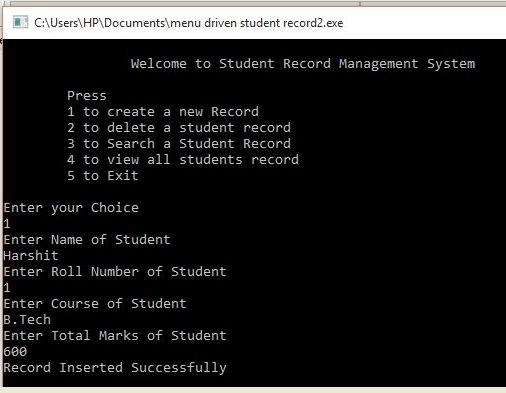• Show Record: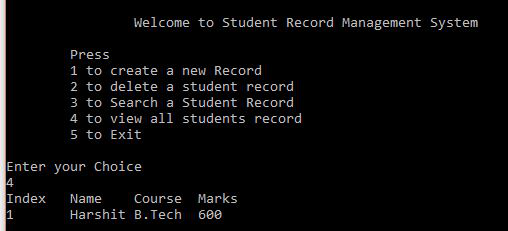• Delete Record: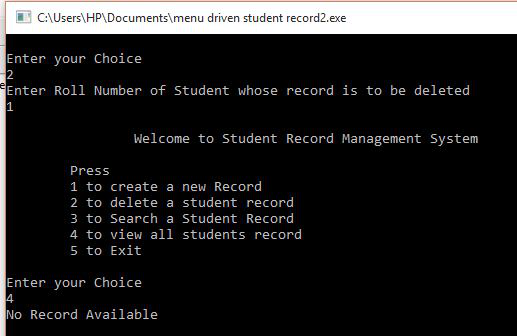• Search Record: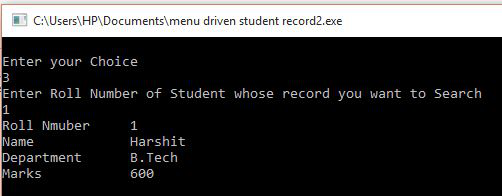• Student Record: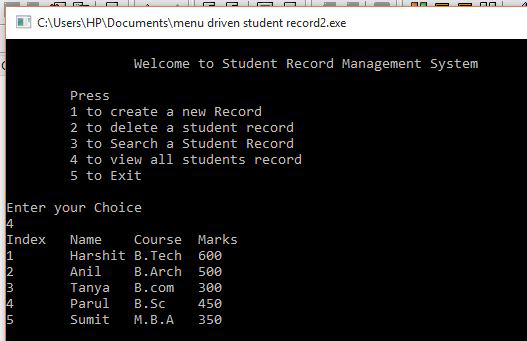My Personal Notes arrow_drop_up